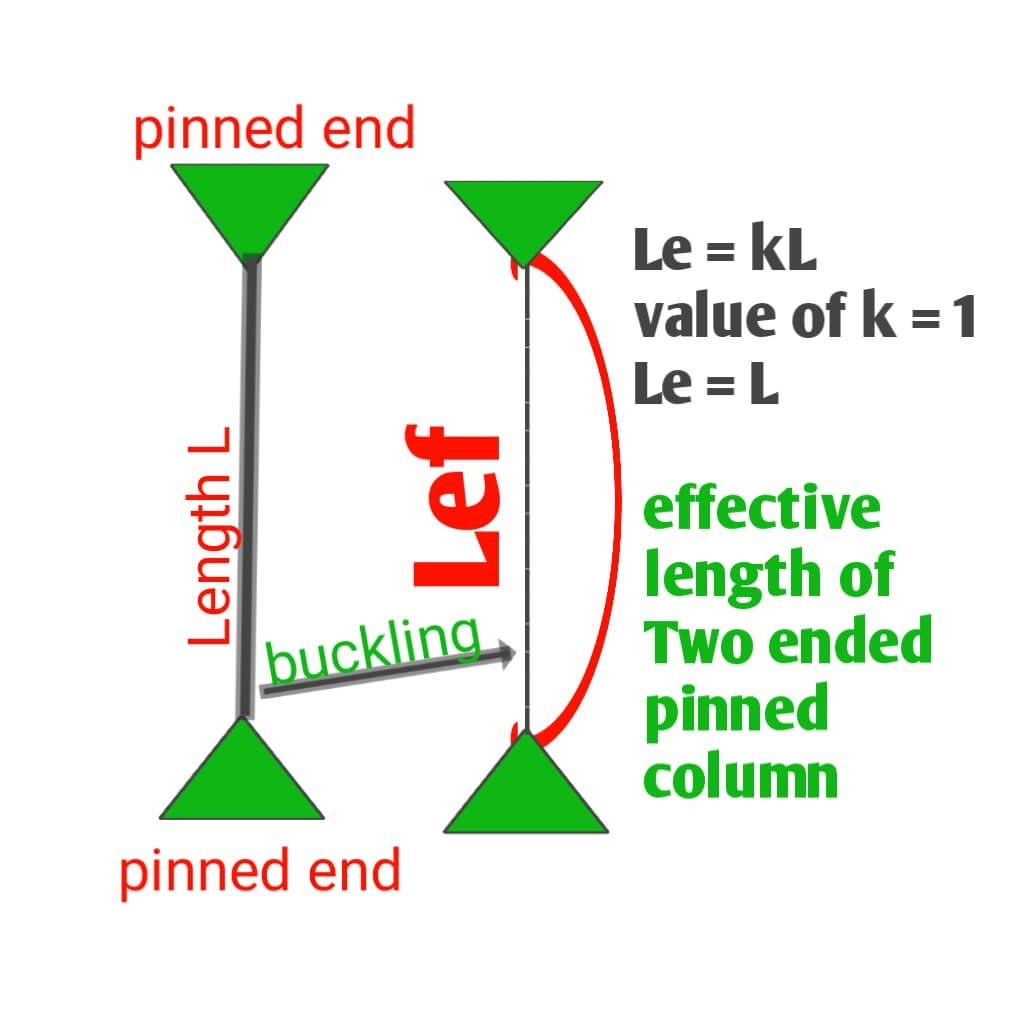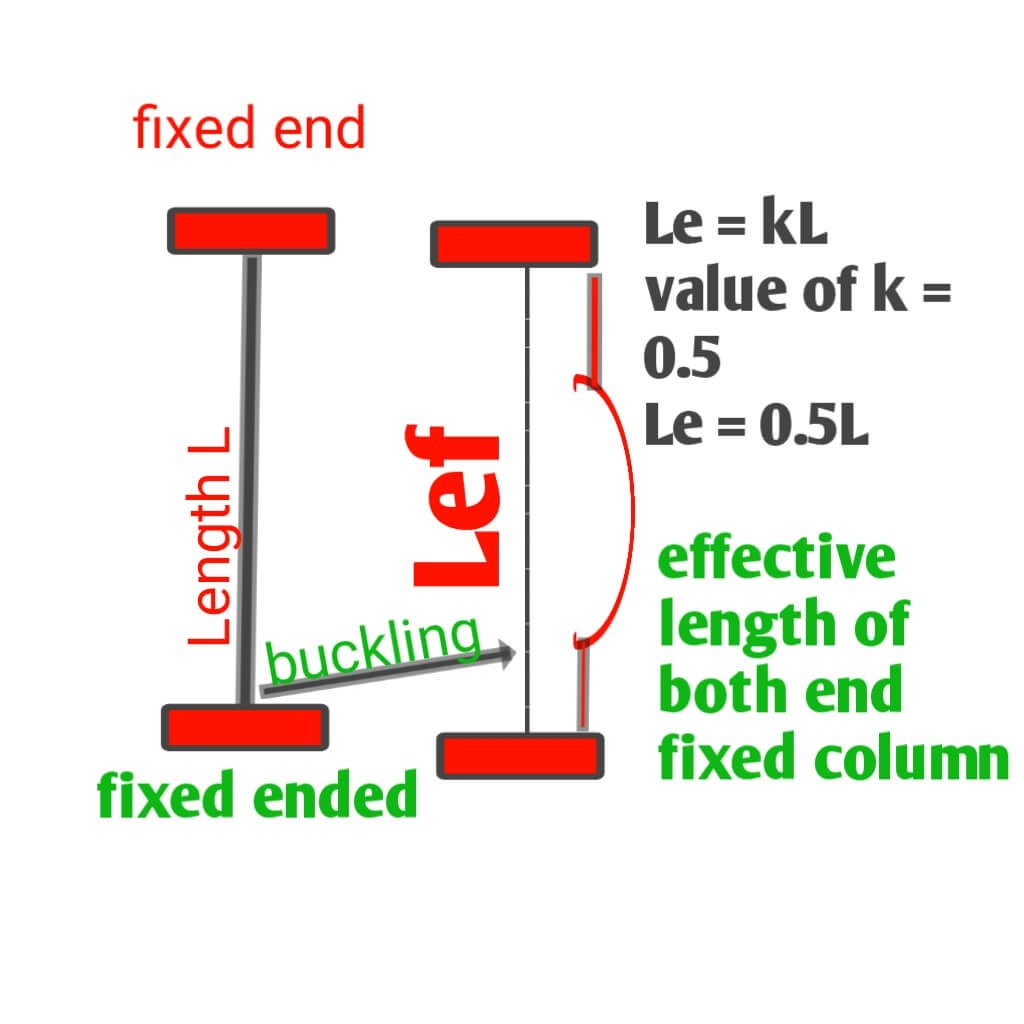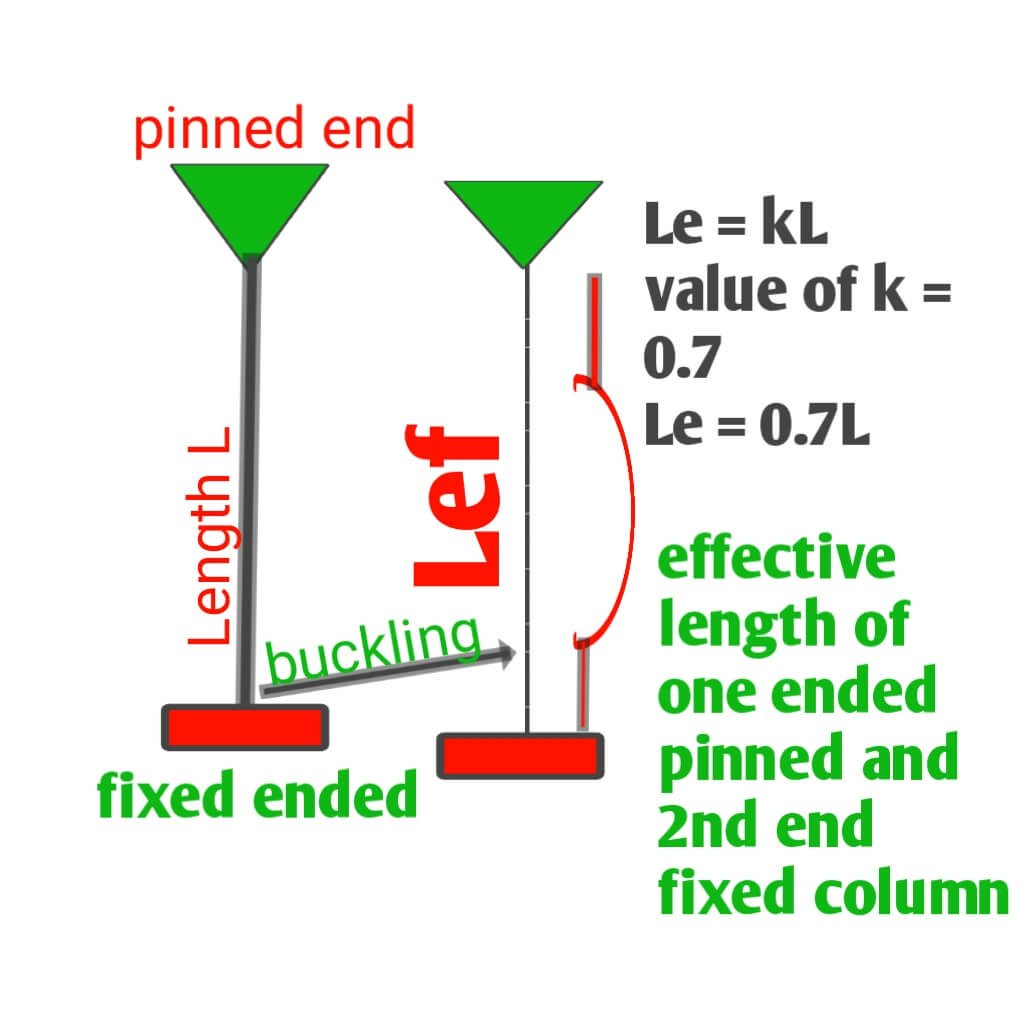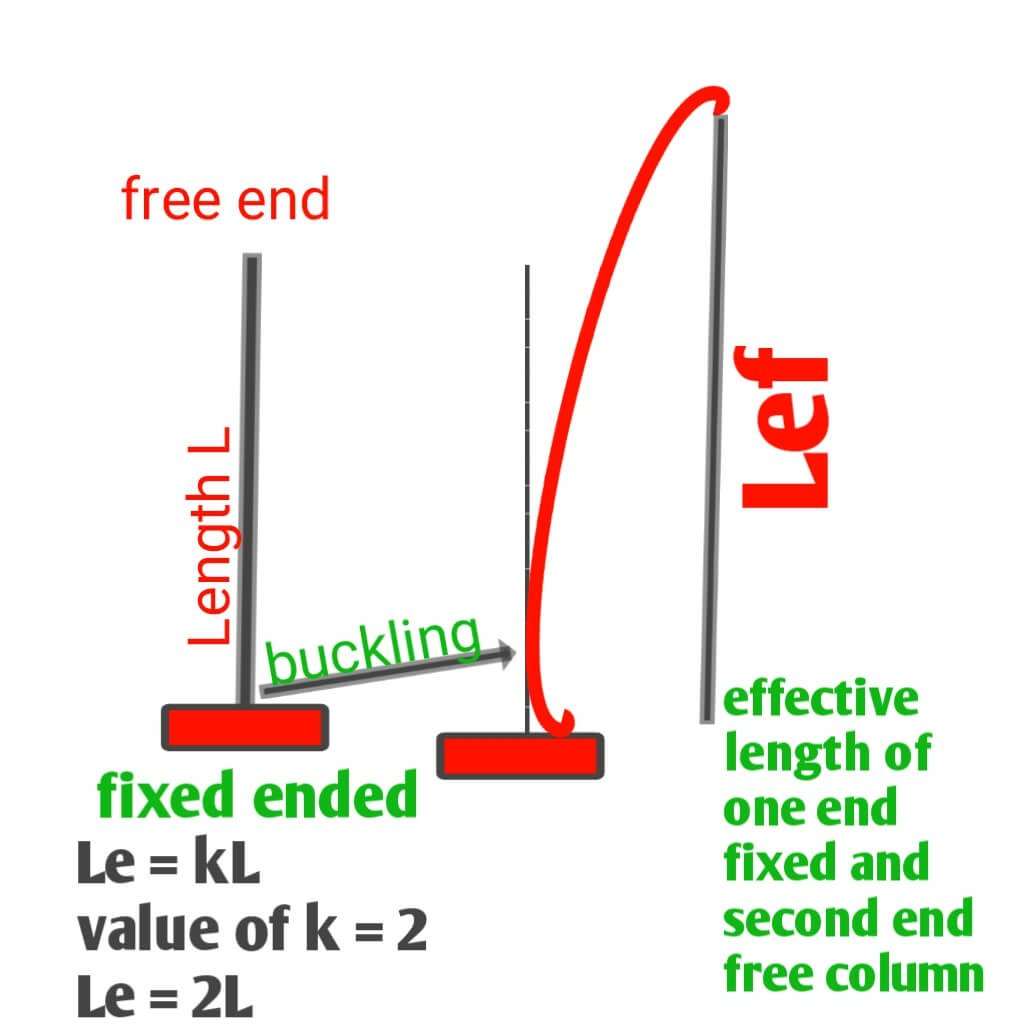# Determination of effective length of column

Determination of effective length of column

Introduction :- hi guys in this article we know about determination of effective length of column. As we know that building construction is framed structure of system have different members such as Foundation column beam and slab. structural column are the member which are spanned across the full height of a structure as well as below the ground and column it crucial part of load transfer mechanism in which all the dead load and live load which is coming from slab and beam acting vertically on column which transfer to the bed of soil.Determination of effective length of column

You should also visits:-

1)what is concrete and its types and properties

2) concrete quantity calculation for staircase and its formula

## What is length of column

The length of column is a vertical distance between two Floor or between two tie level. As structural point of view column in the distance between two point where a column get its fixidity of support with beam for slab. Suppose we have

Length of column = L

## What is effective length of column

Structurally effective length of column is defined as vertical height between the two points of contraflexure of the buckled column or it can be also defined as vertical distance between to deflection caused due to buckling of column. We have two types of column structure first is short column .

column in which the ratio of effective length to least lateral dimension is less than 12 then it is short column and generally short column is fail by compressive stress or crushing. the height in less, so their load carrying capacity is more.

And long column in which the ratio of effective length to least lateral dimension is more than 12 then it is called as Long column. And long column is subject to buckling of beam due to compressive stress. And the height is more so their load carrying capacity in less than short column.

And there is various types of column according to their fixidityEffective length of both end is pinned or hinged column

1) effective length of both end is pinned or hinged column :- the long column which is pinned at both end and free to move and rotate around hinged support but restrain against all transitional movement. When axial load is applied on vertically hinge point of long column resulting compressive stress and column will buckled in entire length

If the the height of column is = L

effective length = kL

Value of k = 1

Than their effective length (Lef ) is equal to height of long column in both ended pinned condition

So Lef = L ,if two end are pinnedeffective length of both fixed ended column

2) effective length of both fixed ended column :- this type of column have two fixed ended that is firmly attached to beam structure and that do not rotate.When axial load is applied on vertically fixed point of long column resulting compressive stress and column will buckled in mid of short length

If the the height of column is = L

effective length = k L

And Value of k = 0.5

Than their effective length (Lef ) is equal to half of height of long column in both ended fixed condition

So Lef = 0.5 L ,if two end are fixedeffective length of one end fixed and second end pinned column

3) effective length of one end fixed and second end pinned column :- in this type of column one end is formally fixed which is not rotate and second end is pinned which is rotate at hinged point.
When axial load is applied on vertically fixed point of long column resulting compressive stress and column will buckled in more than half of length

If the the height of column is = L

effective length = k L

And Value of k = 0.7

Than their effective length (Lef ) is equal to more than half of height of long column in one ended fixed and 2nd end pinned condition

So Lef = 0.7 L ,if one end is fixed and second end is pinnedeffective length of one end fixed and second end free

4) effective length of one end fixed and second end free :- in this type of column One end is fixed which is formally attached to support and second end is free which is freely subject to rotate creating large moment.When axial load is applied on vertically fixed point of long column resulting compressive stress and column will buckled in twice of length

If the the height of column is = L

effective length = k L

And Value of k = 2

Than their effective length (Lef ) is equal to twice of height of long column in one end fixed and 2nd end is free

So Lef = 2 L ,if one end is fixed and 2nd end is free